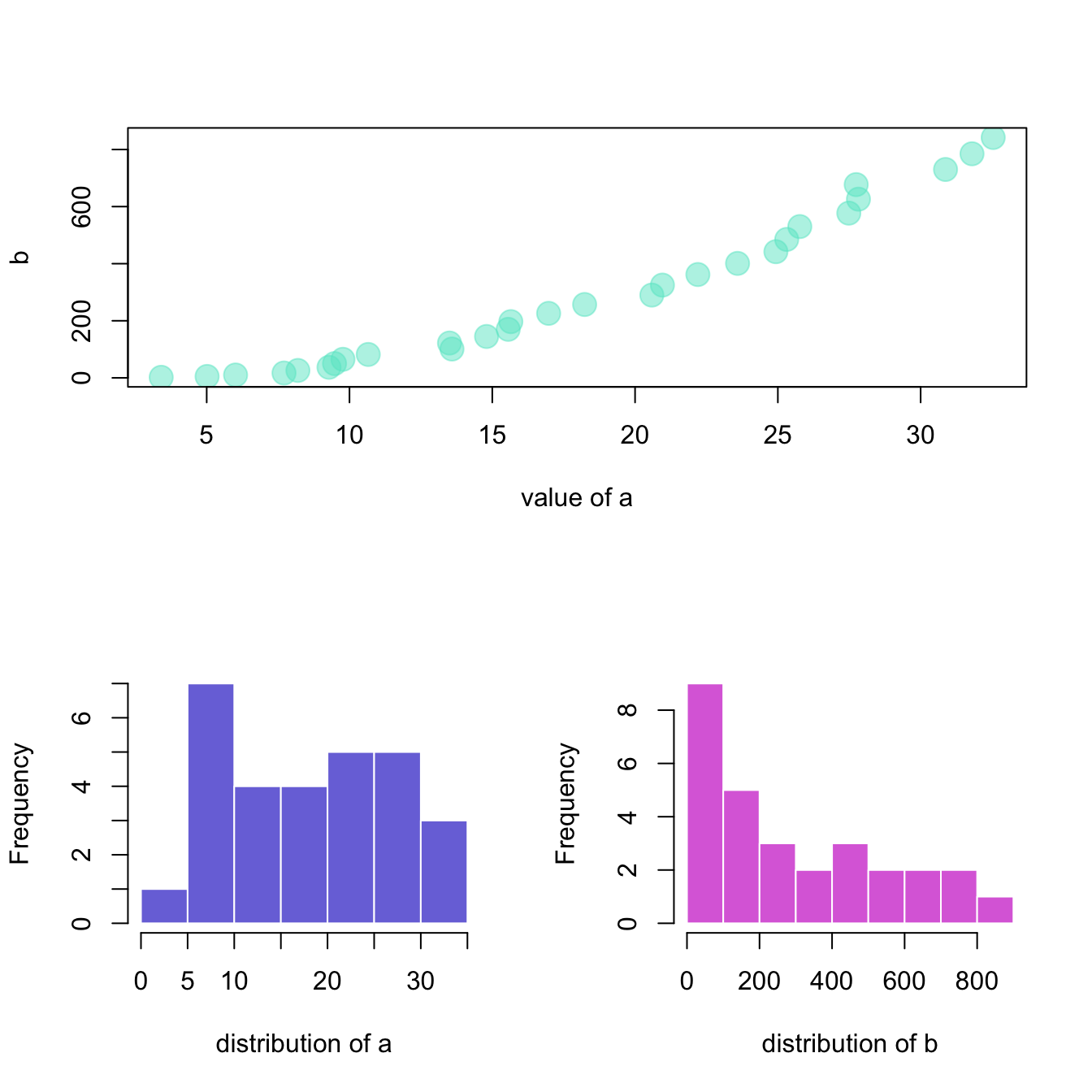# The split_screen() function of R

This document explains how to use the `split_screen()` function of R to divide your device in several parts, one for each chart.

The `split_screen()` function allows to divide the window in several chart sections.

However, I personnaly believe that``````#Create data
a <- seq(1,29)+4*runif(29,0.4)
b <- seq(1,29)^2+runif(29,0.98)

# I divide the screen in 2 line and 1 column only
my_screen_step1 <- split.screen(c(2, 1))

# I add one graph on the screen number 1 which is on top :
screen(my_screen_step1)
plot( a,b , pch=20 , xlab="value of a" , cex=3 , col=rgb(0.4,0.9,0.8,0.5) )

# I divide the second screen in 2 columns :
my_screen_step2 <- split.screen(c(1, 2), screen = my_screen_step1)
screen(my_screen_step2)
hist(a, border=F , col=rgb(0.2,0.2,0.8,0.7) , main="" , xlab="distribution of a")
screen(my_screen_step2)
hist(b, border=F , col=rgb(0.8,0.2,0.8,0.7) , main="" ,  xlab="distribution of b")``````

Related chart types

## Contact

This document is a work by Yan Holtz. Any feedback is highly encouraged. You can fill an issue on Github, drop me a message on Twitter, or send an email pasting yan.holtz.data with gmail.com.